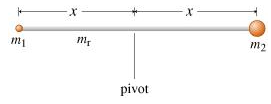# Problem: What is the moment of inertia I of this assembly about the axis through which it is pivoted?Express the moment of inertia in terms of mr, m1, m2, and x. Keep in mind that the length of the rod is 2x, not x.

###### FREE Expert Solution

Moment of inertia of a rod,

$\overline{)\begin{array}{rcl}{\mathbf{I}}& {\mathbf{=}}& \frac{\mathbf{M}{\mathbf{L}}^{\mathbf{2}}}{\mathbf{12}}\end{array}}$

Moment of inertia of a point mass,

$\overline{)\begin{array}{rcl}{\mathbf{I}}& {\mathbf{=}}& {{\mathbf{MX}}}^{{\mathbf{2}}}\end{array}}$

Rod's moment of inertia,

88% (415 ratings)###### Problem DetailsWhat is the moment of inertia I of this assembly about the axis through which it is pivoted?

Express the moment of inertia in terms of mr, m1, m2, and x. Keep in mind that the length of the rod is 2x, not x.